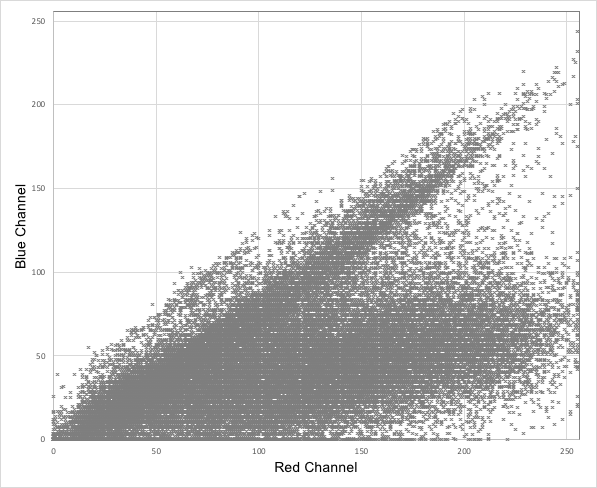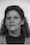## Part 1A: Build Scatter Plots for Color Channels in Image Pixels

Build three scatter plots that describe distributions of pixel values in the following example image. First, update the course app in the VM to version 0.9.8 or later using the 'Update App' desktop shortcut. Second, convert the image to CSV using the 'Images to CSV' tool within the app. Third, use Excel to generate the three scatter plots of color channel values over the image pixels, i.e., red versus green, red versus blue, and green versus blue.

 Input image:Example scatter plot (red vs blue):Insert your scatter plot for red vs blue:Insert your scatter plot for red vs green:Insert your scatter plot for green vs blue:## Part 1B: Compute Mean Vector and Covariance Matrix

Compute the mean vector and the covariance matrix for the color channels over the pixels in the example image used in Part 1A. You can solve this problem using the course app or compute the results using Excel's functions AVERAGE and COVAR.

Fill the missing values in the mean vector:

 Red Green Blue ? 63.68 ?
Fill the missing values in the covariance matrix:

 Red Green Blue Red 3288.89 ? 1082.09 Green ? ? ? Blue ? 1144.04 ?

## Part 1C: Compute Eigenvalues and Eigenvectors

Compute the eigenvectors and the eigenvalues of the covariance matrix from Part 1B. Sort the eigenvalues and the corresponding eigenvectors in increasing order. Use the results to complete the following table.

 1 2 3 Eigenvalue: ? 788.76 ? Red: ? ? ? Green: ? ? ? Blue: ? ? ?

## Part 1D: Find Mahalanobis Distances Between Several Pixels

Compute the Mahalanobis distances between the following four pixels assuming that they were drawn from the statistical distribution of pixels specified by the image from Part 1A. Pixel 1 is black, i.e., all three color channels are zero. Pixel 2 is red, i.e., R=255, G=0, and B=0. Pixel 3 is green, i.e., R=0, G=255, and B=0. Finally, pixel 4 is blue, i.e., R=0, G=0, and B=255.

Fill the missing values in the following matrix formed by the Mahalanobis distances. In this case, the Mahalanobis distance should be computed using the formula d(x, y) = SQRT((x - y)T S-1 (x - y)). Unlike the definition of the Mahalanobis distance in the textbook, in this case the formula uses the square root. This minor modification makes the special case when the covariance matrix is identity equivalent to the regular Euclidean distance.

 Pixel 1 Pixel 2 Pixel 3 Pixel 4 Pixel 1 0 ? 28.09 ? Pixel 2 ? ? ? ? Pixel 3 ? ? 0 ? Pixel 4 ? 15.04 ? ?

## Part 2A: Describe Meaning of a Term in Some Formula

The following formula describes the ratio of the volume of a hypersphere with the radius r to the volume of a hypercube with edges of length 2r. It is often used to illustrate the properties of spaces with many dimensions and is related to the curse of dimensionality. Describe the meaning of the term Γ(d/2) in this formula. How is this term related to the factorial? How is it related to Leonhard Euler? What is the value of Γ(n) if n is a positive integer? If z is a complex number that has a strictly positive real part, then Γ(z) is equal to the Euler integral of which kind?```Insert your answers here. ```

## Part 2B: Explain Notation Often Used in Textbook

The textbook often uses two parallel bars to denote the norm of a vector. Assuming that a and b are two vectors in n-dimensional Euclidean space, give the formula that expresses the values of ||a - b||, ||a||, and ||b|| using the square root function and the three dot products a·a, a·b, and b·b? Finally, assuming that n>1 and that both a and b are column vectors, express ||a - b||2 in terms of a, aT, b, and bT.

 ||a - b|| = Insert your answer that uses the square root function and the three dot products a·a, a·b, and b·b here. ||a|| = Insert your answer here. ||b|| = Insert your answer here. ||a - b||2 = Insert your answer in terms of a, aT, b, and bT here.

## Part 3A: Run PCA on Fisher's Iris Dataset

Run the principal component analysis on Fisher' Iris dataset (using this version). Insert the mean vector, the eigenvalues, and the corresponding eigenvectors into the table below. Also, visualize the dataset in 2D using a scatter plot of the first principal component score versus the second principal component score. Use different markers to indicate the three different classes of instances in the dataset. Preferably, your plot should appear in full color. Also, provide a CSV or Excel file with the component scores that were used to generate the scatter plot.

Fill the missing values in the mean vector:

 Sepal length: Sepal width: Petal length: Petal width: ? 3.06 ? ?

Complete the following table with the eigenvalues and the corresponding eigenvectors of the covariance matrix, starting from the largest eigenvalue.

 1 2 3 4 Egenvalue: ? 0.24 ? 0.02 Sepal length: ? ? ? ? Sepal width: ? ? ? ? Petal length: ? ? ? ? Petal width: ? ? ? ?

 Example scatter plot in grayscale:Insert your scatter plot here:## Part 3B: Train One-vs-Rest Linear SVM on Two PC Scores

Update the course app in the VM to version 0.9.9.1 or later using the 'Update App' desktop shortcut. Then, use the linear SVM tool in the app to determine the equations of three lines that separate each of the three classes in the Iris dataset from the remaining two classes in the space of the first two principal component scores generated in Part 3A. One line should clearly split the leftmost cluster. Another line should clearly split the rightmost cluster. The third line that corresponds to the middle cluster should pass roughly horizontally through the dataset because the middle cluster cannot be easily separated from the other two by only one line.

Use the coefficients for the three separating lines to replace the question marks below:

 Equation for Iris setosa: ? x PC1 + ? x PC2 + ? = 0 Equation for Iris versicolor: ? x PC1 + ? x PC2 + ? = 0 Equation for Iris virginica: ? x PC1 + ? x PC2 + ? = 0

## Part 4A: Train Linear SVM on Wine Dataset

Update the course app in the VM to version 0.9.9.1 or later using the 'Update App' desktop shortcut. Then, train a linear SVM on the wine dataset and state the equation for the hyperplane that separates the two classes. Use the following two files for training: wine_features.csv and wine_labels.csv (they can be downloaded in the VM by updating the datasets). Hint: the weights for the features are stored as the list coef in the linear SVM model CSV file. The value of w0 is called the intercept.

Use the coefficients for the separating hyperplane to replace the question marks below:

 ? x fixed acidity + ? x volatile acidity + ? x citric acid + ? x residual sugar + ? x chlorides + ? x free sulfur dioxide + ? x total sulfur dioxide + ? x density + ? x pH + ? x sulphates + ? x alcohol + ? = 0

## Part 4B: Implement Linear SVM Decision Function in Excel

Use the hyperplane coefficients from Part 4A to implement the linear SVM classification in Excel. Then, measure its accuracy on the training set, i.e., compare the labels predicted for the instances in wine_features.csv with the ground truth in wine_labels.csv and find the percentage of correctly predicted labels (it should be close to 98% or higher).

Fill the missing values in the mean vector:

 Accuracy: ? Number of errors: ? Number of correctly predicted labels: ?

## Part 5A: Visualization Inspired by Eigenfaces

Use PCA on the space of pixels in grayscale to visualize several low-resolution pictures of faces in a 2D grid where the two axes correspond to the scores for the first two principal components (the required computation is similar to what the Eigenfaces algorithm does). Use any program that can position graphics over other graphics, e.g., Microsoft PowerPoint, to arrange images on the scatterplot to indicate which points correspond to which images. First, update the course app in the VM to version 0.9.8 or later using the 'Update App' desktop shortcut. Second, convert the image to CSV using the 'Images to CSV' tool within the app. Finally, use the resulting CSV files to perform PCA and the visualiztion. The input data is given in the following table:An example greyscale figure that should look similar to your results is shown below.Insert your plot to replace the placeholder above.

## Part 5B: Extend Visualization to Images Outside Training Set

Extend the visualization developed in Part 5A to the two new images that weren't used for training without re-running PCA, i.e., by re-using the mean vector and the eigenvectors from Part 5A. The two additional faces are shown in the following table:An example greyscale figure that should look similar to your results is shown below.Insert your plot to replace the placeholder above.